# How to Make A Cell Value as Worksheet Tab Name in Excel

Usually we often define worksheet tab name depending on the contents of this worksheet like Summary, Product List etc. But in some cases, we want to define the worksheet name equals to the text in specified cell, for example we want to the contents in A1 cell is also displayed as the worksheet tab name, how can we do? This article will help you solve this problem by edit VBA code.

## Make Worksheet Tab Name Equals to Cell Value in Excel

In a new excel worksheet, the default tab name is Sheet1. We can rename it by directly type a new name to replace it. We can also define tab name as same as the value in cell A1 (or any other cell you like) by VBA. See details below.

Step 1: Right click on Sheet1 to load Sheet management menu. Select View Code, Microsoft Visual Basic for Applications window pops up.

Or you can enter Microsoft Visual Basic for Applications window via Developer->Visual Basic.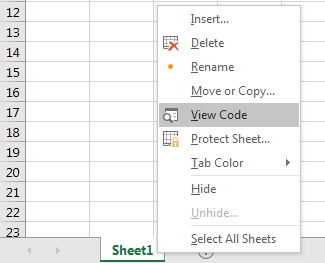Step 2: In Microsoft Visual Basic for Applications window, click on Sheet1, enter below code:

```Private Sub Worksheet_Change(ByVal Target As Range)

ActiveSheet.Name = ActiveSheet.Range("A1")

End Sub```

Step 3: Save the codes. And quit Microsoft Visual Basic for Applications.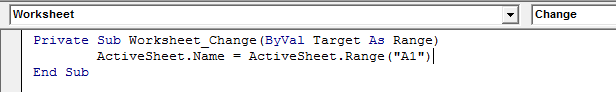Step 4: In current sheet1, in cell A1, enter the tab name for example Product List, then click Enter.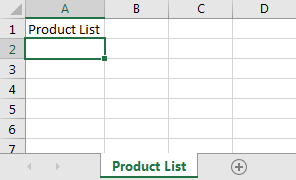Verify that Sheet1 is changed to Product List which is saved in cell A1.

Notes:

This code is only applied for worksheet1. So, for other worksheets it doesn’t work.

If enter a value with invalid characters like /, error message will pop up. See screenshot below: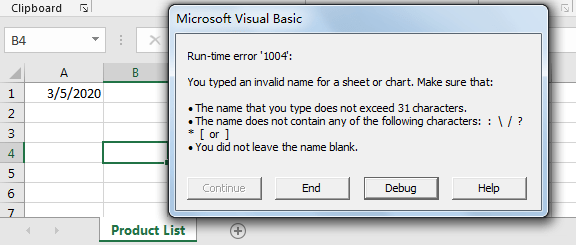If A1 is blank or clear A1, below error message will pop up.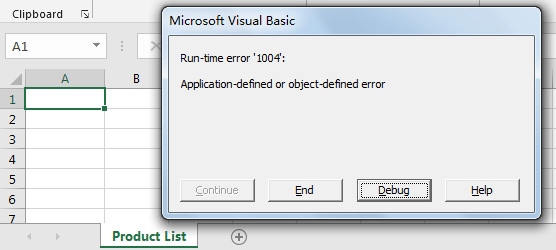So, if we want avoid the case of blank A1, we can edit code with more criteria:

```Private Sub Worksheet_Change(ByVal Target As Range)

If Not Intersect(Target, Range("A1")) Is Nothing Then

ActiveSheet.Name = ActiveSheet.Range("A1")

End If

End Sub```

You can change A1 cell to other cell by replace A1 in above code.

Related Posts

Find and Replace Multiple Values

This post will guide you how to find and replace multiple values at once with VBA macro or using formula  in Excel. How do I make multiple find and replace in Excel. Suppose that you have a few cells containing ...

VBA Macro For VLOOKUP From Another Sheet

In the previous post, you should know that how to fix or remove the #N/A error when using VLOOKUP formula to lookup value from another sheet. And this post will show you how to use VBA code to vlookup data ...

How To Insert Comments in Protected Worksheet in Excel

This post will show you how to allow comments in a protected worksheet in Excel. You can easily to insert comments into cells in a normal worksheet in Excel, but if want to insert a comment in a worksheet that ...

How To Convert Text to Upper Cases(Using VBA) in Excel

This post will show you how to switch from lower case to upper case in Excel. and I am going to show you two different ways of converting text to upper cases using formula or VBA macro in Excel 2013,Excel ...

How To Hide Every Other Row in Excel (Using VBA)

This post will show you how to hide alternate rows or columns in Excel or how to hide every third, fourth, fifth row or column in Excel. If you want to hide every other row in your current worksheet, how ...

How to Disable the Save As Prompt in Excel

This post will show you how to use a VBA Macro to save an Excel file and overwrite any existing file without a prompt so that you are going to get the little window that says file already exists do ...

How to Count Cells that Contain even or odd numbers in Excel

This post will guide you how to count the number of cells that contain odd or even numbers within a range of cells using a formula in Excel 2013/2016.How do I count cells that contain odd numbers through the use ...

How to Count Cells that Contain negative Numbers in Excel

This post will guide you how to count the number of cells that contain negative numbers within a range of cells using a formula in Excel 2013/2016.You can count the number of negative numbers in your data using easy functions ...

How to Count Cells Are Not Blank or Empty in Excel

This post will guide you how to count cells that are not blank or empty in a given range cells using a formula in Excel 2013/2016.How do I count the number of cells that are not blank in a particular ...

How to Count Cells Less Than a Specific Value in Excel

This post will guide you how to count the number of cells less than a particular numeric value in a given range cells using a formula in Excel 2013/2016. How do I count cells that are less than a specific ...

Sidebar